# RS Aggarwal Class 9 Solutions Chapter 6 - Coordinate Geometry

## RS Aggarwal Class 9 Chapter 6 - Coordinate Geometry Solutions Free PDF

Coordinate Geometry is an important branch of Geometry, which tells us the position of points on a plane with the help of ordered pairs of numbers called coordinates. It is also known as Analytic or Cartesian geometry. All the points in coordinate geometry are located on a plane for easy understanding, this plane is called a coordinate plane.

The various applications of Coordinate Geometry are:

1. Space Science
2. Rocketry
3. Space Flight
4. Differential Geometry
5. Computational Geometry
6. Aviation

## Download PDF of RS Aggarwal Class 9 Solutions Chapter 6 – Coordinate Geometry

Solve questions from RS Aggarwal Class 9 solutions for better understanding and practice it on a regular basis to have a strong grasp of all the concepts mentioned in the RS Aggarwal Class 9 maths textbook. These solutions will help you to perform well in your examination and can be referred to whenever you have any doubt.

QUESTION 1: Write down the coordinates of each of the points A, B, C, D, E and F shown below draw :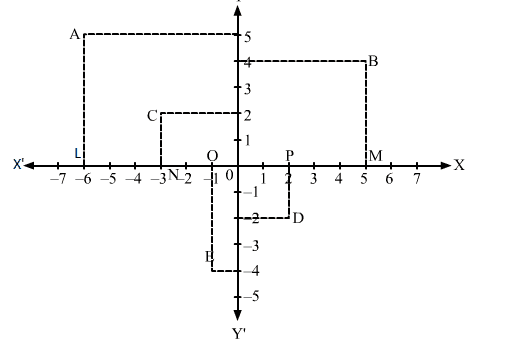SOLUTION:

Draw the perpendiculars from the AF, BG, CH, DI, and EJ on the x-axis:

1) The distance of A from the x-axis= OF= -6 units
The distance of A from y-axis =AF =5 units
Hence, the coordinate of A is (-6, 5)

2) The distance of B from the x-axis= OG= 5 units
The distance of B from y-axis = BG = 4 units
Hence, the coordinate of B is (5, 4)

3) The distance of C from the x-axis= OH= -3 units
The distance of C from y-axis = HC =2 units
Hence, the coordinate of C is (-3, 2)

4) The distance of D from the x-axis= OI= 2 units
The distance of D from y-axis = ID = -2 units
Hence, the coordinate of D is (-3, -2)

5) The distance of C from the x-axis= OJ= -1 units
The distance of C from y-axis = JE = -4 units
Hence, the coordinate of A is (-1, -4)

Thus, the coordinates of A, B, C, D, and E are respectively:

A (-6, 5), B (5, 4) , C (-3, 2) and E (-1, -4)

QUESTION 2 : Draw the lines X’OX and Y’OY as the coordinate Axes on a paper and plot the following points on it.

i. P (7, 4)

ii. Q (-5, 3)

iii. R (-6, -3)

iv. S (3, -7)

v. A (6, 0)

vi. B (0, 9)

vii. O (0, 0)

vii. C (-3, -3)

SOLUTION:

Let X’OX and Y’OY be the co-ordinate axes.

Fix the side of the small squares as one units:

i. Starting from O, take +7 units on the x-axis and then +4 units on the y-axis to obtain the point P (7, 4).

ii. Starting from O, take -5 units on the x-axis and then +3 units on the y-axis to obtain the point P (-5, 3).

iii. Starting from O, take -6 units on the x-axis and then -3 units on the y-axis to obtain the point P (-6, -3).

iv. Starting from O, take 3 units on the x-axis and then -7 units on the y-axis to obtain the point P (3, -7).

v. Starting from O, take 6 units on the x-axis and then 0 units on the y-axis to obtain the point P (6, 0).

vi. Starting from O, take 0 units on the x-axis and then 9 units on the y-axis to obtain the point P (0, 9).

vii. Starting from O, take 0 units on the x-axis and then 0 units on the y-axis to obtain the point P (0, 0).

viii. Starting from O, take -3 units on the x-axis and then -3 units on the y-axis to obtain the point P (-3, -3).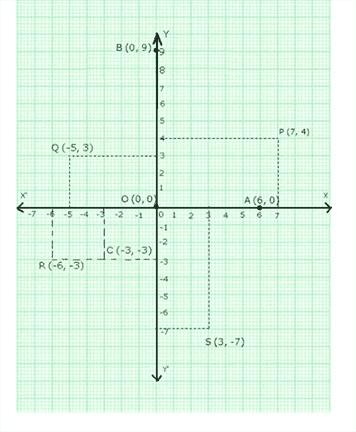QUESTION 3 : On which axes did the following points lie?

1. (7, 0)

2. (0, -5)

3. (0, 1)

4. (-4, 0)

SOLUTION:

1. In (7, 0) we have the ordinate = 0

Therefore, (7, 0) lies on the x-axis

2. In (0, -5), we have the abscissa = 0

Therefore, (0, -5) lies on the y-axis

3. In (0, 1), we have the abscissa = 0

Therefore, (0, 1) lies on the y-axis

4. In (-4, 0), we have the ordinate = 0

Therefore, (-4, 0) lies on the x-axis

QUESTION 4 : In which quadrant do the given points lie?

1. (-6, 5)

2. (-3, -2)

3. (2, -9)

SOLUTION:

1. Point of the type (-, +) lies in the 2nd quadrant.

Therefore, the point (-6, 5) lies in the II quadrant.

2. Point of the type (-, -) lie in the 3rd quadrant.

Therefore, the point (-3, -2) lies in the III quadrant.

3. Point of the type (+, -) lies in the 4th quadrant.

Therefore, the point (2, -9) lies in the IV quadrant.

QUESTION 5 : Draw the graph of the equation, y = x + 1

SOLUTION:

The given equation is y = x + 1

Putting x =1, we get y = 1 + 1 = 2

Putting x =2, we get y = 2 + 1 = 3

Thus, we have the following table:

 x 1 2 Y 2 3

On a graph paper, draw the lines X’OX and Y’OY as the x-axis and y-axis respectively.

Now, plot points P (1, 2) and Q (2, 3) on the graph paper.

Join PQ and extend it in both the directions.

Then, line PQ is the graph of the equation y = x + 1.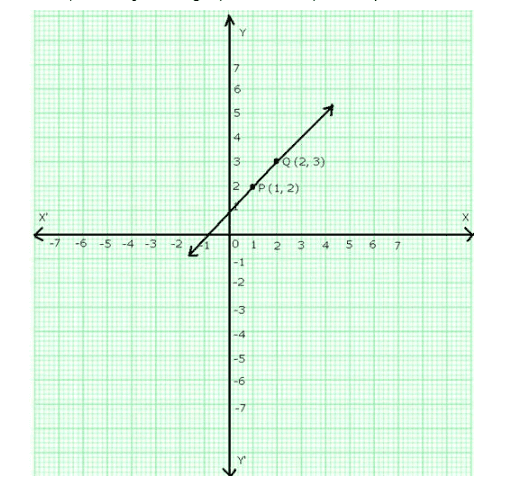QUESTION 6 : Draw the graph of the equation, y = 3x + 2

SOLUTION:

The given equation is y = 3x + 2

Putting x = 1, we get y = (3 * 1) + 2 = 5

Putting x = 2, we get y = (3 * 2) + 2 = 8

Thus, we have the following table:

 X 1 2 Y 5 8

On a graph paper draw the lines X’OX and Y’OY as the x-axis and y-axis respectively.

Now, plot points P (1, 5) and (2, 8).

Join PQ and extend it in both the directions.

Then, line PQ is the graph of the equation y = 3x + 2.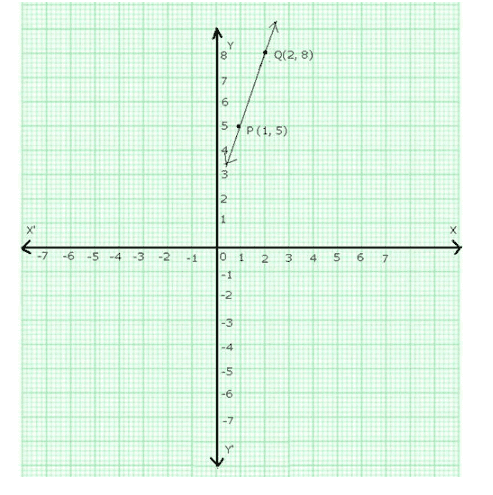QUESTION 7 : Draw the graph of the equation, y = 5x – 3

SOLUTION:

The given equation is y = 5x – 3

Putting x = 0, we get y = (5 * 0) – 3 = – 3

Putting x = 1, we get y = (5 * 1) -3 = 2

Thus, we have the following table:

 X 0 1 Y -3 2

On a graph paper draw the lines X’OX and Y’OY as the x-axis and y-axis respectively.

Now, plot points P (0, -3) and (1, 2).

Join PQ and extend it in both the directions.

Then, line PQ is the graph of the equation y = 5x – 3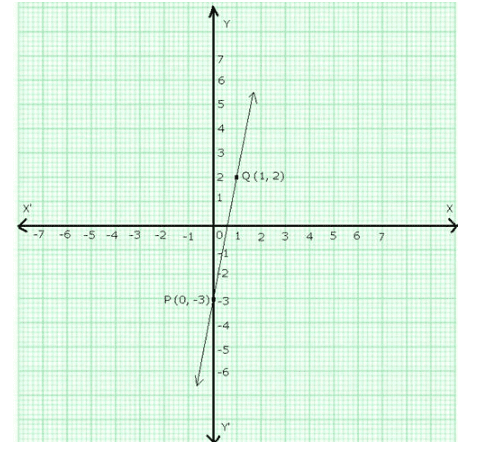QUESTION 8 : Draw the graph of the equation, y = 3x

SOLUTION:

The given equation is y = 3x

Putting x =1, we get y = (3, 1) = 3

Putting x =2, we get y = (3, 2) = 6

Thus, we have the following table:

 X 1 2 Y 3 6

On a graph paper draw the lines X’OX and Y’OY as the x-axis and y-axis respectively.

Now, plot points P (1, 3) and (2, 6)

Join PQ and extend it in both the directions.

Then, line PQ is the graph of the equation y = 3x.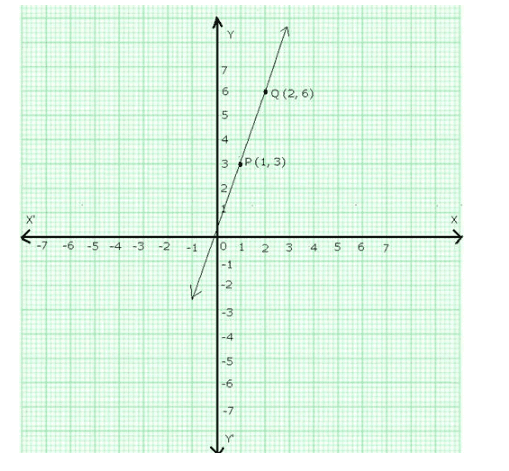QUESTION 9 : Draw the graph of the equation, y = – x

SOLUTION:

The given equation is y = 3x

Putting x =1, we get y = (3 * 1) = 3

Putting x =2, we get y = (3 * 2) = 6

Thus, we have the following table:

 X 1 2 Y -1 -2

On a graph paper draw the lines X’OX and Y’OY as the x-axis and y-axis respectively.

Now, plot points P (1, -1) and (2, -2).

Join PQ and extend it in both the directions.

Then, line PQ is the graph of the equation y =  -x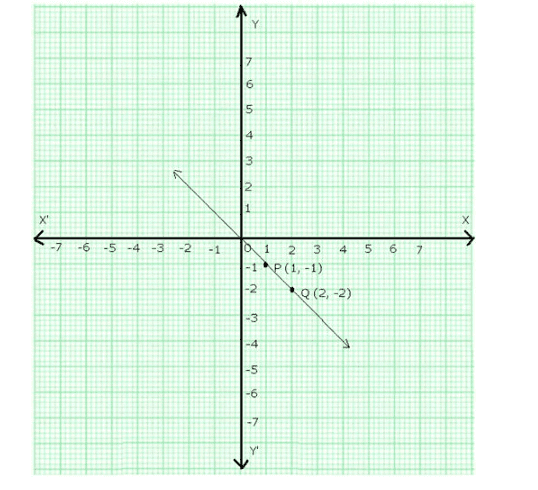### RS Aggarwal Class 9 Solutions Chapter 6 – Coordinate Geometry

To help students to score good marks in their Class 9 exam we have provided the complete and accurate RS Aggarwal Maths Solution for Chapter 6 designed by our subject experts based on the latest syllabus of Class 9. All the solutions are equipped with a perfect solving method of the questions mentioned in the RS Aggarwal Class 9 maths textbook. These solutions will help you to have a deeper understanding of problems which will definitely help to analyze your performance.